• 利用Qt5.7自带的QChart实现极坐标绘图，同时实现封闭曲线内部填充颜色，实现角度坐标轴旋转，解决坐标旋转后，坐标Label显示多行的问题，实现多个R方向显示坐标Label。
• ## MATLAB之极坐标绘图

万次阅读 2018-07-09 14:57:47
阅读数：5134转发：...说到极坐标绘图，第一个想到的就是polar啦~那就先试试吧！1.polar绘图polar函数用来绘制极坐标图，调用格式为：polar（theta，rho，选项）其中，the...
阅读数：5134转发：https://blog.csdn.net/Sumujingling/article/details/50884209目标是要绘制一个二维的极坐标彩色图。输入参数有三个，一个是角度，一个是半径，一个是颜色。说到极坐标绘图，第一个想到的就是polar啦~那就先试试吧！1.polar绘图polar函数用来绘制极坐标图，调用格式为：polar（theta，rho，选项）其中，theta为极坐标极角，rho为极径，选项的内容和plot函数相似。例：绘制 的极坐标图clcclear allclose alltheta = 0:0.01:2*pi;rho = sin(2*theta).*cos(2*theta);figurepolar(theta,rho,'--r')polar不能满足我的需求！我要绘制的不是曲线，是面，嗷！附赠一个好玩的例程~！a=-2*pi:.001:2*pi; %设定角度b=(1-sin(a)); %设定对应角度的半径polar(a, b,'r') %绘图2.contour由于polar不能满足我的需求，我就在matlab里面help了一下，在polar plots 目录下看到一个有趣的例程图片来自contour。于是准备试试。先跑一个例程：theta = 0:0.01:2*pi;rho = sin(2*theta).*cos(2*theta);[TH, R] = meshgrid(theta,rho);[X,Y] = pol2cart(TH, R);Z = X +1i*Y;f = (Z.^4-1).^(1/4);figurecontour(X,Y,abs(f),30);axis equal;xlabel('Real');ylabel('Imaginary');结果美如画：感觉有点想我想要的呀~赶紧深入研究一下。然而事实是残酷的。为什么我上传的图片都不显示！！！
展开全文• 极坐标绘图函数polar 格式： polar(theta,rho,LineSpec); %theta：与X轴正半轴的夹角，为弧度；rho：与原点的距离 角度转换函数 格式： 弧度=degtorad(角度); %将角度从度数转换为弧度 极坐标转化为直角坐标 格式...
极坐标绘图0 角度转换函数degtorad1 极坐标绘图1.1 极坐标绘图函数1.2 极坐标图添加注释1.3 更改极坐标区范围1.4 极坐标半径、角度、网格设置2 坐标系转换2.1 极坐标转化为直角坐标2.2 直角坐标转化为极坐标
0 角度转换函数degtorad
弧度=degtorad(角度); %将角度从度数转换为弧度

1 极坐标绘图
1.1 极坐标绘图函数
♡polar函数
polar(theta,rho,LineSpec);
%theta：与X轴正半轴的夹角，为弧度；rho：与原点的距离

♡polarplot函数
polarplot(theta,rho);
%theta是用弧度制表示的角度，rho是对应的半径

绘制多个极坐标线图，可以使用hold on保留当前极坐标区，然后通过 polarplot绘制其他数据图。
1.2 极坐标图添加注释
legend('滤波前','滤波后');
title('减去名义半径轮廓形状');

1.3 更改极坐标区范围
默认情况下，在极坐标图中半径的负值将被绘制为正值。使用rlim将r坐标轴范围调整为包含负值。
rmin=min(rho);
rmax=max(rho);
rlim([rmin rmax]);

使用thetalim将theta坐标轴范围更改为0到180。
thetalim([0 180]);

1.4 极坐标半径、角度、网格设置
set(gca,'RAxisLocation',5); %设置半径坐标轴位置
set(gca,'RTick',[-1 -0.5 0 0.5 1],'RTickLabel',{'-1','-0.5','0','0.5','1'}); %设置极坐标半径
set(gca,'ThetaTick',[0 30 60 90 120 150 180 210 240 270 300 330],'ThetaTickLabel',{'0°','30°','60°','90°','120°','150°','180°','210°','240°','270°','300°','330°'}); %设置极坐标角度
set(gca,'GridLineStyle','--','GridAlpha',0.6); %设置网格线型、粗细

2 坐标系转换
2.1 极坐标转化为直角坐标
函数pol2cart
[x,y]=pol2cart(theta,rho);
[x,y,z]=pol2cart(theta,rho,z);
%x=rho*cos(theta);
%y=rho*sin(theta);

2.2 直角坐标转化为极坐标
函数cart2pol
[theta,rho]=cart2pol(x,y);
[theta,rho,z]=cart2pol(x,y,z);
%theta=atan2(y,x);
%rho=sqrt(x.^2+y.^2);



展开全文matlab
• 极坐标绘图 // An highlighted block import numpy as np import matplotlib.pyplot as plt r=np.arange(1,6) #角度变化 theta=[0,np.pi/2,np.pi,3*np.pi/2,2*np.pi] ax=plt.subplot(111,projection='polar') #...
文章目录极坐标绘图正方形绘图三角形绘图
极坐标绘图
// An highlighted block
import numpy as np
import matplotlib.pyplot as plt
r=np.arange(1,6)
#角度变化
theta=[0,np.pi/2,np.pi,3*np.pi/2,2*np.pi]
ax=plt.subplot(111,projection='polar')
#（theta：角度，r：绘图点的位置，linewidth：线条宽度）
ax.plot(theta,r,color='r',linewidth=3)
ax.grid(True)
plt.show()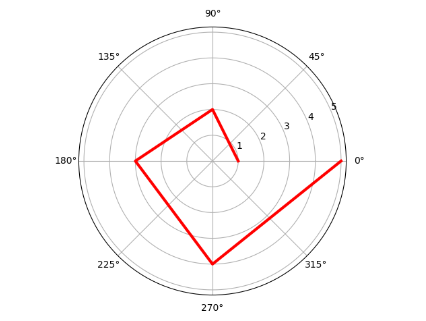正方形绘图
// An highlighted block
import numpy as np
import matplotlib.pyplot as plt
r=np.arange(5)
#将r只取5
r.fill(5)
#0,90,180,270,360
theta=[0,np.pi/2,np.pi,3*np.pi/2,2*np.pi]
ax=plt.subplot(111,projection='polar')
ax.plot(theta,r,color='r',linewidth=3)
ax.grid(True)
plt.show()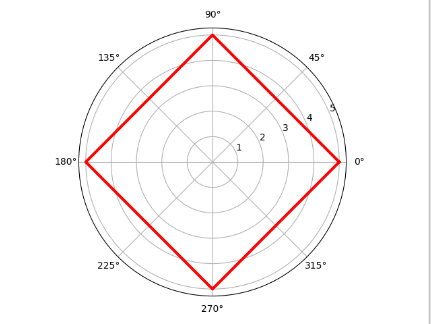三角形绘图
// An highlighted block
import numpy as np
import matplotlib.pyplot as plt
r=np.arange(4)
r.fill(4)
#-30,90,210,330
theta=[-np.pi/6,np.pi/2,7*np.pi/6,11*np.pi/6]
ax=plt.subplot(111,projection='polar')
ax.plot(theta,r,color='r',linewidth=3)
ax.grid(True)
plt.show()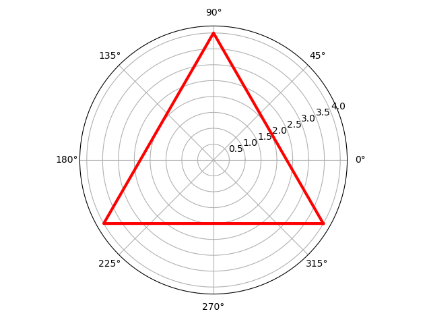展开全文可视化
• Polar Chart 极坐标图绘制
文章目录一、Polar Chart 极坐标图问题二、Polar Chart 极坐标图

一、Polar Chart 极坐标图问题

应一位博客粉丝提出的问题 , 给出 MATLAB 绘图方案 ;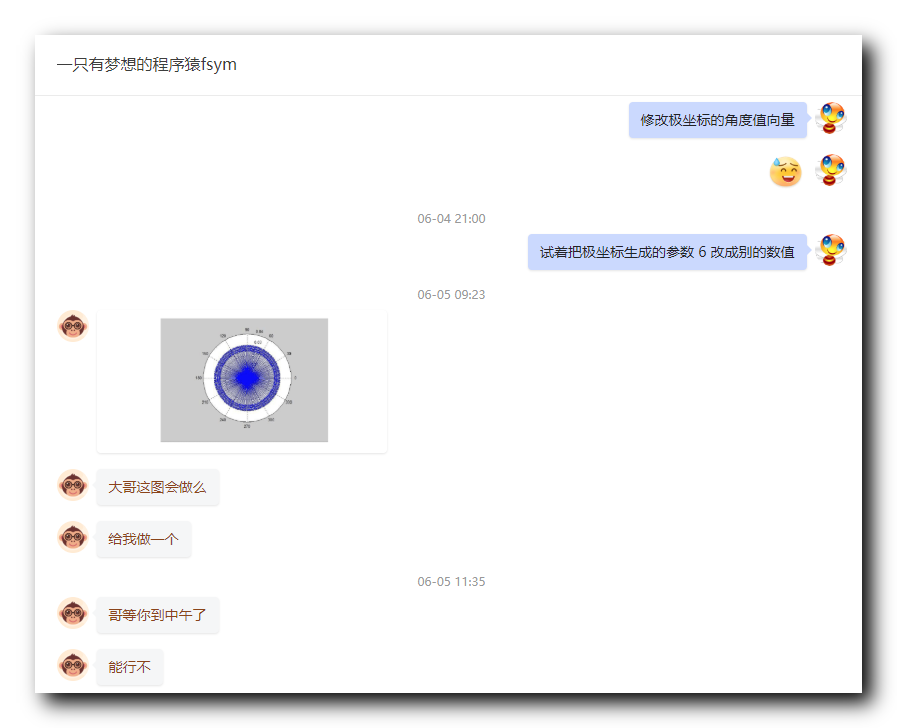绘图样式 :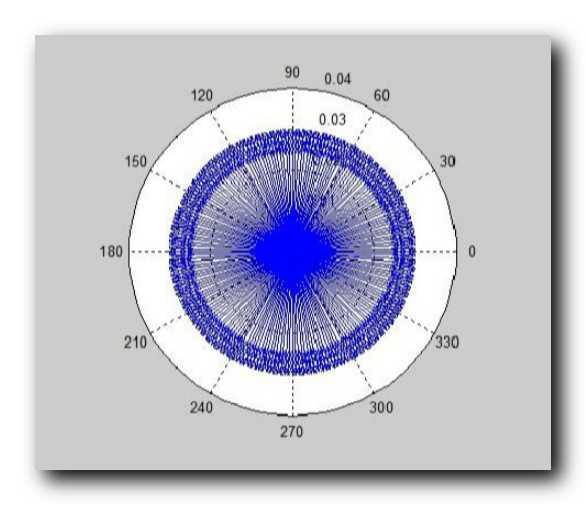二、Polar Chart 极坐标图

首先极坐标范围是 $0$ ~ $0.04$ , 绘制的第一个曲线 , 先标定范围 , 用于限定最大值 ;
% 绘制极坐标图, 设置极坐标的最大值范围
polar(0, 0.04);

当前绘制效果 : 仅用于限定坐标系范围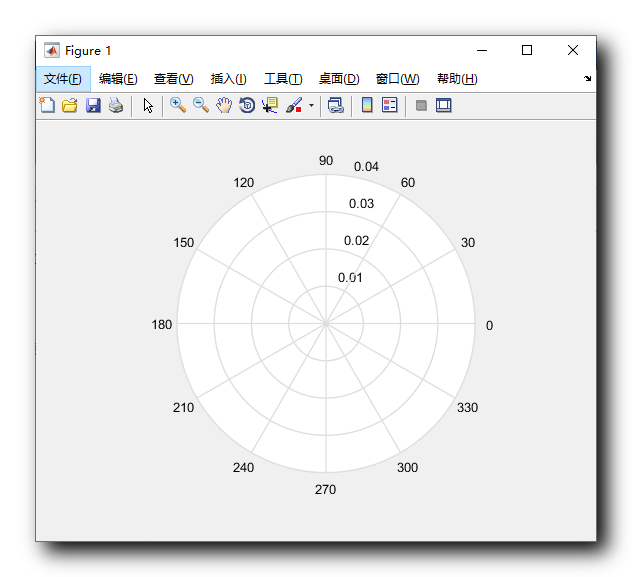绘制内层稀疏曲线 :
为了使曲线看起来更加平滑 , 这里设置角度采样的个数 $100000$ , 样本个数越多 , 曲线越平滑 ,
半径向量值直接图中是 $0.03$ , 直接使用该向量值 ;
将两个曲线绘制在一个极坐标系中 , 这样需要在两个 polar 函数之间使用
hold on;

代码 ;
设置曲线绘制颜色在 polar 函数中 , 第三个参数使用 'b' 指定 , 绘制曲线是蓝色的 ;
polar(theta, r, 'b');

当前阶段代码 :
% 角度值向量
theta = linspace(0, 2 * pi, 100000);
% 半径值向量
r = 0.03 * cos(60 * theta);

% 绘制极坐标图, 设置极坐标的最大值范围
polar(0, 0.04);

hold on;

% 绘制内层图像
polar(theta, r, 'b');

当前绘制效果 :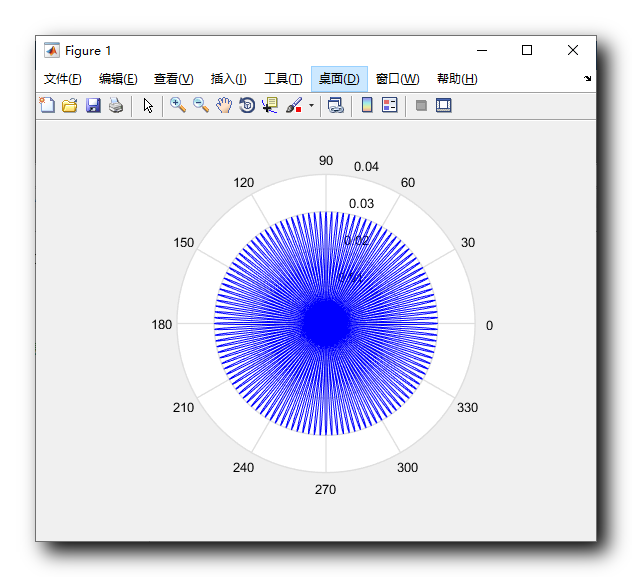绘制外层密集曲线 :
外层曲线的角度值范围也是 $0$ ~ $2 \pi$ ,
只是半径的向量在 $0.027$ ~ $0.03$ 之间 , 这里直接使用 $0.027 + 0.03 \times \cos(250 * \rm theta1)$  获得 , 设置 $250$ 是因为需要获得更加密集的曲线坐标点 ;
% 角度值向量
theta1 = linspace(0, 2 * pi, 100000);
% 半径值向量
r1 = 0.027+ 0.003 * cos(250 * theta1);

将两个曲线绘制在一个极坐标系中 , 这样需要在两个 polar 函数之间使用
hold on;

代码 ;
设置曲线绘制颜色在 polar 函数中 , 第三个参数使用 'b' 指定 , 绘制曲线是蓝色的 ;
polar(theta1, r1, 'b');

完整绘图代码 :
% 角度值向量
theta = linspace(0, 2 * pi, 100000);
% 半径值向量
r = 0.03 * cos(60 * theta);

% 角度值向量
theta1 = linspace(0, 2 * pi, 100000);
% 半径值向量
r1 = 0.027+ 0.003 * cos(250 * theta1);

% 绘制极坐标图, 设置极坐标的最大值范围
polar(0, 0.04);

hold on;

% 绘制内层图像
polar(theta, r, 'b');

hold on;

% 绘制外层图像
polar(theta1, r1, 'b');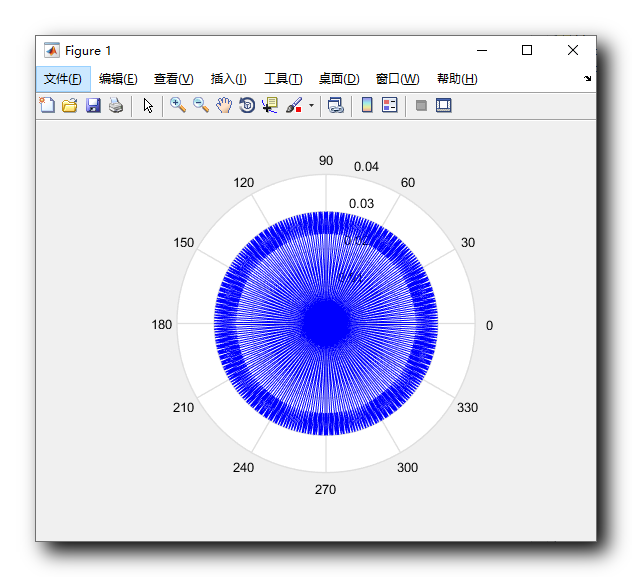如果要求改绘图的曲线密度 , 修改
% 半径值向量
r = 0.03 * cos(60 * theta);

和
% 半径值向量
r1 = 0.027+ 0.003 * cos(250 * theta1);

中 $\cos$ 函数内的值 , 即 $60$ 和 $250$ 这两个值 ;


展开全文matlab polar
• MATLAB三维绘图不论是surf还是mesh都是在笛卡尔坐标中进行，没有专门的三维极坐标绘图。以天线三维方向图为例，先将极坐标转换为笛卡尔坐标，然后使用surf在MATLAB中绘制，与HFSS图形对比，得到一致结果
• 从理解原理入手，使用matplotlib绘制你想要的...# 除了直角坐标系，还有极坐标系，两个维度分别表示角度（弧度）与模长 # 设置方法非常简单，只需要在定义axes对象时指定projection参数为'polar'即可 from matplotli...
• 这是笔者的个人博客，包含以下内容： 1 常用命令 标注 子图绘制 图片保存 ...极坐标绘图 函数绘图 等高线地图 3 三维绘图 三维条形图 三维散点图 4 其他 制作 gif 访问网址 Matlab-drawing ...matlab 经验分享
• 另外在医学仪器上面，X光的CT扫描也会使用极坐标，因为它也是采用旋转发射，然后再接收的方式实现，非常适合极坐标进行绘图。可见，极坐标在生活中使用非常广泛，也极其有用，像医院里CT扫描检测，已经作为主要分析...python matplotlib 数据分析
• 前面学习了极坐标来显示数据，接着下来学习更多的极坐标显示的例子，这样对于以后想显示更多的方式，提供了有价值的参考。 比如在极坐标里绘画散点图： 在这个例子里，主要是下面这行代码： fig, ax = plt....python matplotlib 数据可视化...